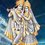# Don't know how to solve. Do you?

$Q.1$ Find all quadruples $(a,b,c,d)$ such that : $(b+c+d)^{2010}$=$3a$ ;
$(a+c+d)^{2010}$=$3b$ ;
$(b+a+d)^{2010}$=$3c$ ;
$(b+c+a)^{2010}$=$3d$ ;

$Q.2$ In an acute triangle $ABC$ , the segment $CD$ is an altitude and $H$ is orthocentre. Given that circumcentre of triangle lies on angle bisector of angle $DHB$, determine possible values of angle $CAB$.Note by Aakash Khandelwal
5 years, 4 months ago

This discussion board is a place to discuss our Daily Challenges and the math and science related to those challenges. Explanations are more than just a solution — they should explain the steps and thinking strategies that you used to obtain the solution. Comments should further the discussion of math and science.

When posting on Brilliant:

• Use the emojis to react to an explanation, whether you're congratulating a job well done , or just really confused .
• Ask specific questions about the challenge or the steps in somebody's explanation. Well-posed questions can add a lot to the discussion, but posting "I don't understand!" doesn't help anyone.
• Try to contribute something new to the discussion, whether it is an extension, generalization or other idea related to the challenge.

MarkdownAppears as
*italics* or _italics_ italics
**bold** or __bold__ bold
- bulleted- list
• bulleted
• list
1. numbered2. list
1. numbered
2. list
Note: you must add a full line of space before and after lists for them to show up correctly
paragraph 1paragraph 2

paragraph 1

paragraph 2

[example link](https://brilliant.org)example link
> This is a quote
This is a quote
    # I indented these lines
# 4 spaces, and now they show
# up as a code block.

print "hello world"
# I indented these lines
# 4 spaces, and now they show
# up as a code block.

print "hello world"
MathAppears as
Remember to wrap math in $$ ... $$ or $ ... $ to ensure proper formatting.
2 \times 3 $2 \times 3$
2^{34} $2^{34}$
a_{i-1} $a_{i-1}$
\frac{2}{3} $\frac{2}{3}$
\sqrt{2} $\sqrt{2}$
\sum_{i=1}^3 $\sum_{i=1}^3$
\sin \theta $\sin \theta$
\boxed{123} $\boxed{123}$

Sort by:

Here is the diagram:Triangle ABC
$O$ is the circumcentre of $\triangle ABC$.
Now, do you know these properties? They are simple to prove, so if you don't, I can show you how.
1. $\angle DHB = A$
2. $\angle DHA = B$
3. $\angle OAB = \frac{\pi}{2} - C$
4. $\angle HAB = \frac{\pi}{2} - B$
5. $HA = 2R \cos A$ where $R = OA$ is the circumradius.

From these we get,
$\angle HAO = \angle HAB - \angle OAB = C - B$
$\angle OHA = \angle DHA + \angle DHO = \frac{A}{2} + B$

So I'm aiming for a sine rule, but I need $\angle HOA$ for that.
We have $\angle HOA = \pi - (\angle HAO + \angle OHA$)

Now in $\triangle AHO$,
$\dfrac{2R \cos A}{\sin \angle HOA} = \dfrac{R}{\sin \angle OHA}$

Using the values we've got,
$2 \cos A = \dfrac {\sin (\frac{A}{2} + C)}{\sin (\frac{A}{2} + B)}$
$\dfrac {\sin (\frac{A}{2} + C)}{\sin (\frac{A}{2} + B)} = \dfrac {2 \sin (\frac{A}{2} + C) \cos \frac{A}{2}}{2 \sin (\frac{A}{2} + B)\cos \frac{A}{2}} = \dfrac{\sin (A + C) + \sin C}{\sin (A + B) + \sin B}$

But, $A + C = \pi - B$ and $A + B = \pi - C$
So, $\sin (A + C) = \sin B$ and $\sin (A + B) = \sin C$

$\dfrac{\sin (A + C) + \sin C}{\sin (A + B) + \sin B} = \dfrac{\sin B + \sin C}{\sin C + \sin B} = 1$

So, $2 \cos A = 1$ and $A = \frac{\pi}{3}$

- 5 years, 4 months ago

Awesome!! Try to get first also.

- 5 years, 4 months ago

A faster way (sigh, how did I miss this?) would be to note that, $(\frac{A}{2} + B) + (\frac{A}{2} + C) = \pi$
and hence, $\sin(\frac{A}{2} + B) = \sin(\frac{A}{2} + C)$

- 5 years, 4 months ago

Note that $\angle DHB = \angle A$. Use the sine rule and then trig bash. I'm searching for a nicer solution.

EDIT: Turns out I was using the wrong triangle. Use triangle $HOA$ where $O$ is the circumcentre. The angles are all nice. You get $A = \pi/3$. Will post full solution tomorrow.

- 5 years, 4 months ago

I think the answer to first is $a=b=c=d=0$ or $a=b=c=d=1/3$

- 5 years, 4 months ago

Yeah, you're right. Sorry, I solved the question, but didn't post the solution.
This is just an outline:
1. Since the conditions are symmetric, assume $a \geq b \geq c \geq d \geq e$ without loss of generality.
2. Then use the conditions given to show equality for all variables. (But I think it should be mentioned that $a, b, c, d, e$ are positive.)

- 5 years, 4 months ago

No , it wasn't mentioned from where I picked it up

- 5 years, 3 months ago